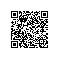# 【DB 2学习】查看一个数据库的配置文件

db2 => get db cfg for sample
数据库 sample 的数据库配置
数据库配置发行版级别                                    = 0x0c00
数据库发行版级别                                        = 0x0c00

数据库地域                                              = CN
数据库代码页                                            = 1208
数据库代码集                                            = UTF-8
数据库国家/地区代码                                    = 86
数据库整理顺序                                          = IDENTITY
备用整理顺序                              (ALT_COLLATE) =
数字兼容性                                              = OFF
Varchar2 兼容性                                         = OFF
数据库页大小                                            = 8192

动态 SQL 查询管理                      (DYN_QUERY_MGMT) = DISABLE

对此数据库的发现支持                      (DISCOVER_DB) = ENABLE

限制访问                                                = NO
缺省查询优化类                           (DFT_QUERYOPT) = 5
并行度                                     (DFT_DEGREE) = 1
在算术异常时继续                      (DFT_SQLMATHWARN) = NO
缺省刷新有效期                        (DFT_REFRESH_AGE) = 0
缺省维护的选项（DFT_MTTB_TYPES）的表类型                = SYSTEM
保留的高频值的数目                     (NUM_FREQVALUES) = 10
保留的分位点数目                        (NUM_QUANTILES) = 20

十进制浮点舍入方式                    (DECFLT_ROUNDING) = ROUND_HALF_EVEN
备份暂挂                                                = NO
数据库是一致的                                          = NO
前滚暂挂                                                = NO
复原暂挂                                                = NO
启用的多页文件分配                                      = YES
恢复状态的日志保留                                      = RECOVERY
日志记录状态的用户出口                                  = YES
自调整内存                            (SELF_TUNING_MEM) = OFF
数据库共享内存大小（4KB）             (DATABASE_MEMORY) = AUTOMATIC
数据库内存阈值                          (DB_MEM_THRESH) = 10
锁定列表的最大存储量（4KB）                   (LOCKLIST) = 50
每个应用程序的锁定百分比列表                 (MAXLOCKS) = 22
程序包高速缓存大小（4KB）                  (PCKCACHESZ) = (MAXAPPLS*8)
共享排序的排序堆域值（4KB）            (SHEAPTHRES_SHR) = 5000
排序列表堆（4KB）                            (SORTHEAP) = 256

数据库堆（4KB）                                (DBHEAP) = AUTOMATIC
目录高速缓存大小（4KB）               (CATALOGCACHE_SZ) = (MAXAPPLS*4)
日志缓冲区大小（4KB）                         (LOGBUFSZ) = 8
实用程序堆大小（4KB）                    (UTIL_HEAP_SZ) = 5000
缓冲池大小（页）                             (BUFFPAGE) = 250
SQL 语句堆（4KB）                            (STMTHEAP) = AUTOMATIC
缺省应用程序堆（4KB）                      (APPLHEAPSZ) = AUTOMATIC
应用程序内存大小（4KB）                (APPL_MEMORY) = AUTOMATIC
统计信息堆大小（4KB）                    (STAT_HEAP_SZ) = AUTOMATIC

检查死锁的时间间隔（毫秒）                  (DLCHKTIME) = 10000
锁定超时（秒）                             (LOCKTIMEOUT) = -1

更改的页阈值                           (CHNGPGS_THRESH) = 60
异步页清除程序的数目                   (NUM_IOCLEANERS) = AUTOMATIC
I/O 服务器的数目                        (NUM_IOSERVERS) = AUTOMATIC
索引排序标志                                 (INDEXSORT) = YES
顺序检测标志                                (SEQDETECT) = YES
缺省预取大小（页）                    (DFT_PREFETCH_SZ) = AUTOMATIC

跟踪修改的页数                               (TRACKMOD) = ON

容器的缺省数目                                          = 1
缺省表空间扩展数据块大小(页)            (DFT_EXTENT_SZ) = 32

最大活动应用程序数                           (MAXAPPLS) = AUTOMATIC
活动应用程序的平均数目       (AVG_APPLS) = AUTOMATIC
每个应用程序的最大打开数据库文件数           (MAXFILOP) = 32768

日志文件大小（4KB）                         (LOGFILSIZ) = 1000
主日志文件的数目                           (LOGPRIMARY) = 3
辅助日志文件的数目                          (LOGSECOND) = 2
已更改的至日志文件的路径                   (NEWLOGPATH) =
日志文件路径                                            = D:\DB2\NODE0000\SQL00
002\SQLOGDIR\
溢出日志路径                          (OVERFLOWLOGPATH) =
镜像日志路径                            (MIRRORLOGPATH) =
首个活动日志文件                                        = S0000016.LOG
磁盘上已满的块日志                    (BLK_LOG_DSK_FUL) = NO
事务使用的最大主日志空间的百分比        （MAX_LOG）= 0
1 个活动 UOW 的活动日志文件的数目        (NUM_LOG_SPAN) = 0

组落实计数                                  (MINCOMMIT) = 1
软检查点前回收的日志文件的百分比              (SOFTMAX) = 100
启用的恢复的日志保留                        (LOGRETAIN) = RECOVERY
启用的日志记录的用户出口                     (USEREXIT) = ON

第一个日志归档方法                        (LOGARCHMETH1) = USEREXIT
logarchmeth1 的选项                        (LOGARCHOPT1) =
第二个日志归档方法                        (LOGARCHMETH2) = OFF
logarchmeth2 的选项                        (LOGARCHOPT2) =
故障转移日志归档路径                     (FAILARCHPATH) =
错误时重试日志归档次数                   (NUMARCHRETRY) = 5
日志归档重试延迟（秒）                 (ARCHRETRYDELAY) = 20
供应商选项                                  (VENDOROPT) =

启用的自动重新启动                        (AUTORESTART) = ON
索引重新创建时间和重做索引构建               (INDEXREC) = SYSTEM (RESTART)
要保留的数据库备份的数目               (NUM_DB_BACKUPS) = 12
恢复历史记录保留时间（天数）          (REC_HIS_RETENTN) = 366
自动删除恢复对象                     (AUTO_DEL_REC_OBJ) = OFF

TSM 管理类                              (TSM_MGMTCLASS) =
TSM 节点名                               (TSM_NODENAME) =
TSM 所有者                                  (TSM_OWNER) =

自动维护                                   (AUTO_MAINT) = ON
自动数据库备份                       (AUTO_DB_BACKUP) = OFF
自动表维护                           (AUTO_TBL_MAINT) = ON
自动 runstats                       (AUTO_RUNSTATS) = ON
自动语句统计信息                  (AUTO_STMT_STATS) = OFF
自动统计信息概要分析              (AUTO_STATS_PROF) = OFF
自动概要文件更新                  (AUTO_PROF_UPD) = OFF
自动重组                       (AUTO_REORG) = OFF
启用 XML 字符操作                      (ENABLE_XMLCHAR) = YES
WLM 收集时间间隔（分钟）              (WLM_COLLECT_INT) = 0使用钉钉扫一扫加入圈子
+ 订阅